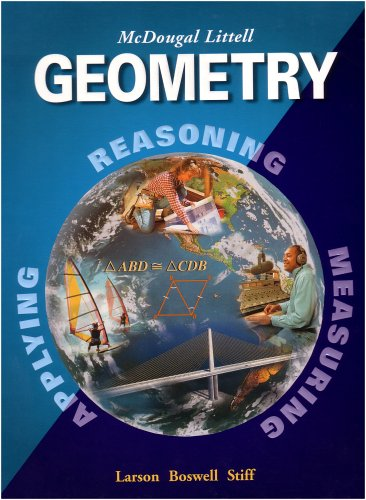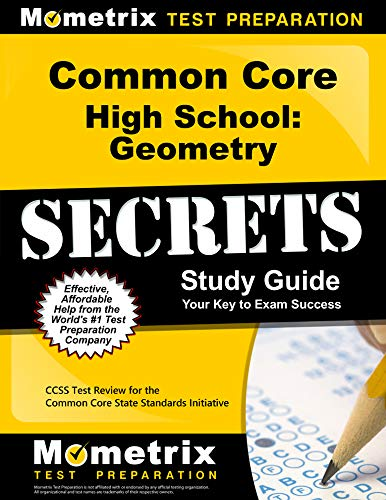# Best Geometry Books for High School | Enhance your Knowledge

Geometry is an important branch of mathematics. Mainly it discusses on shape. Every sphere of life we have to use geometry for various purposes. In robotics, computers, video games, geographic information systems, star maps and space travel, we have to use geometrical knowledge. For this, you must learn geometry from the very basics in high school. These best geometry books article has all the information on geometry to enrich your knowledge for your high school.

## 1. Painless Geometry (Barron's Painless)

This amazing book is a part of painless book series. It's very useful for middle and high school students. It offers all the topics very informally and student-friendly approaches and emphasize on interesting details. Students begin to understand how disparate details all fit together to form a clear picture. Also, you can learn how geometric principles can solve real-world problems. In a word, the best book for improving each student's grades in their geometry courses

What you'll learn-

• Characteristics of distinct shapes

• Real-world problems

• "Brain Tickler" quizzes

• Relationships between parallel and perpendicular lines

• Ideas for interesting projects

## 2. Geometry, Grades 9-12: Mcdougal Littell High School Math (McDougal Littell High Geometry)Author: Ron Larson,Laurie Boswell,Lee Stiff
Published at: 01/01/2004
ISBN: 0618250220

This Geometry textbook is really helpful for students who are planning to take or willing to take PAP Geometry in high school. Formulas and explanations of all aspects of geometry are well explained. Every topic is easy to understand. Also, example questions after each explanation are very essential.

What you'll learn-

• Practice questions

• Online quizzes and tests

• Geometrical theorem

• Applications of Two-Dimensional and Three-Dimensional Geometry

• Explanations on shape and forms

## 3. Tutor In a Book's Geometry

A complete book to guide yourself like a private tutor. It provides you with geometrical explanations, drawing, hint and memory tools. All the topics are decorated in a way that students can easily understand, remember and recognize the connections between geometry and its effects in real life.

What you'll learn-

• More than 500 problems

• Well-illustration of every problem

• Explained proofs

• Test-taking strategies

• Recurring patterns

• Dozens of graphic organizers

## 4. Girls Get Curves: Geometry Takes Shape

A special book for girls which is written by Hollywood actress and math specialist Danica. It shows you how to feel confident and cut good marks in mathematics courses. Also, you can learn effective techniques for high school geometry and explanations of all triangle theorems.

What you'll learn-

• Time-saving tips and tricks

• Homework and tests

• Practice problems with detailed solutions

• Real-world examples

• True stories from Danica’s own life as an actress and math student

• Troubleshooting problems

## 5. The Humongous Book of Geometry Problems (Humongous Books)

This book is for those students who want to learn geometry more and solve geometrical problems. Solutions with missing steps and simplifying concepts can make all the topics perfectly clear to the students. By learning how to interpret and solve problems, students become fully prepared to solve an obscure problem.

What you'll learn-

• 1000 problems with solutions

• Step by step instruction on geometry

• Real-world examples and so on

## 6. Common Core High School: Geometry Secrets Study Guide: CCSS Test Review for the Common Core State Standards InitiativeAuthor: CCSS Exam Secrets Test Prep Team
Published at: 14/02/2013
ISBN: 1627330402

This book includes practice test questions and easy to read essential topics. All the topics given in this book are most important for any high school geometry exam. The book explains the right triangles and trigonometry with all the theorems and practice problems. Not only this, Geometric Measurements and Dimensions are also included in this book. Overall, a perfect book to clarify your geometrical knowledge from the very basic.

What you'll learn-

• Detailed overview of expressing geometric properties with equations

• An analysis of circles

• Comprehensive practice questions

• Proving the Pythagorean Theorem using similar triangles

• The Law of Sines and Cosines

• Solve problems involving right triangles

• Cavalieri's principle

• The formula for the area of a sector

• The formula for the area of a circle

• The formula for the volume of a cone

## 7. Geometry by Construction: Object Creation and Problem-solving in Euclidean and Non-Euclidean Geometries

This book is great for college students for geometrical understanding. Each section provides an introduction, rules for each geometry, guidance on some need-to-know constructions. It covers all the usual topics for geometry proofs for any high school geometry course and gives you a good opportunity to review all the rules. Also, it contains many challenging questions that can help you to think more deeply. You can learn all the geometrical explanations with the help of the Euclidean theory.

What you'll learn-

• Rules for each geometry

• Guidance on some need-to-know constructions

• Proofs for all constructions

• Hyperbolic and elliptic geometry

• Euclidean college geometry

• Non-Euclidean models

Thanks for reading this post. If you have any opinion don't hesitate to comment here. Also please subscribe our newsletter to get more updates.